login  home  contents  what's new  discussion  bug reports help  links  subscribe  changes  refresh  edit

fricas
p ==> 3
Type: Void
fricas
K ==> PrimeField p
Type: Void
fricas
P ==> UnivariatePolynomial('x, K)
Type: Void
fricas
x: P := monomial(1,1)$P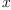(1) Type: UnivariatePolynomial(x,PrimeField?(3)) fricas f: P := (x+2)*(2*x^5 + x^3 + 2)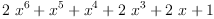(2) Type: UnivariatePolynomial(x,PrimeField?(3)) fricas g: P := 2*x^3 + x^2 -x +1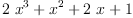(3) Type: UnivariatePolynomial(x,PrimeField?(3)) fricas f/g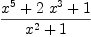(4) Type: Fraction(UnivariatePolynomial(x,PrimeField?(3))) fricas factor f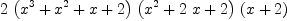(5) Type: Factored(UnivariatePolynomial(x,PrimeField?(3))) fricas factor g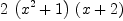(6) Type: Factored(UnivariatePolynomial(x,PrimeField?(3))) fricas k: K := 2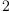(7) Type: PrimeField?(3) fricas f/k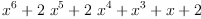(8) Type: UnivariatePolynomial(x,PrimeField?(3)) In order to work with a finite field of order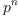where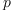is a prime, define the second argument of UnivariatePolynomial as FiniteField?(p, n). fricas F ==> FiniteField(p, 2) Type: Void fricas b: Vector F := basis()$F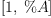(9)
Type: Vector(FiniteField?(3,2))
fricas
t: F := b.2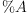(10)
Type: FiniteField?(3,2)
fricas
1+t+t^2+t^3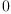(11)
Type: FiniteField?(3,2)
fricas
PF ==> UnivariatePolynomial('y, F)
Type: Void
fricas
y: PF := monomial(1,1)$PF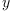(12) Type: UnivariatePolynomial(y,FiniteField?(3,2)) fricas pf: PF := (y+2)*(2*y^5 + y^3 + 2)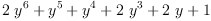(13) Type: UnivariatePolynomial(y,FiniteField?(3,2)) fricas pg: PF := (2*t+1)*y^3 + (1+t)*y^2 -t*y +1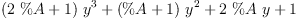(14) Type: UnivariatePolynomial(y,FiniteField?(3,2)) fricas pf/pg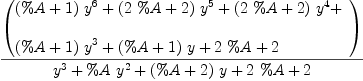(15) Type: Fraction(UnivariatePolynomial(y,FiniteField?(3,2))) fricas factor pf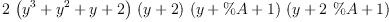(16) Type: Factored(UnivariatePolynomial(y,FiniteField?(3,2))) fricas factor pg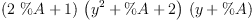(17) Type: Factored(UnivariatePolynomial(y,FiniteField?(3,2))) In case the field property is not needed, IntegerMod? would be the way to go. But note that in general there are zero divisors, so division will not work (even not in the case where the argument of IntegerMod? is a prime). The reason is IntegerMod? doesn't check whether it's argument is prime and so does not know that it might be an integral domain. fricas R ==> IntegerMod(p) Type: Void fricas PR ==> UnivariatePolynomial('z, R) Type: Void fricas z: PR := monomial(1,1)$PR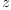(18)
Type: UnivariatePolynomial(z,IntegerMod?(3))
fricas
rf: PR := (z+2)*(2*z^5 + z^3 + 2)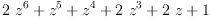(19)
Type: UnivariatePolynomial(z,IntegerMod?(3))
fricas
rg: PR := 2*z^3 + z^2 -z +1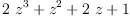(20)
Type: UnivariatePolynomial(z,IntegerMod?(3))
fricas
rf/rg
There are 11 exposed and 15 unexposed library operations named /
having 2 argument(s) but none was determined to be applicable.
Use HyperDoc Browse, or issue
)display op /
package-calling the operation or using coercions on the arguments
will allow you to apply the operation.
Cannot find a definition or applicable library operation named /
with argument type(s)
UnivariatePolynomial(z,IntegerMod(3))
UnivariatePolynomial(z,IntegerMod(3))
Perhaps you should use "@" to indicate the required return type,
or "$" to specify which version of the function you need. Also note that factorization of rf fails. The reason most probably is that the used algorithm needs to divide by some coefficient and it fails to recognize that this is in fact possible (at least for primes p). In IntegerMod?, there simply is no division. fricas r1: R := 2(21) Type: IntegerMod?(3) fricas r2: R := 1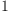(22) Type: IntegerMod?(3) fricas r1/r2 There are 11 exposed and 15 unexposed library operations named / having 2 argument(s) but none was determined to be applicable. Use HyperDoc Browse, or issue )display op / to learn more about the available operations. Perhaps package-calling the operation or using coercions on the arguments will allow you to apply the operation. Cannot find a definition or applicable library operation named / with argument type(s) IntegerMod(3) IntegerMod(3) Perhaps you should use "@" to indicate the required return type, or "$" to specify which version of the function you need.

 Subject:   Be Bold !! ( 14 subscribers )